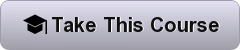Home University Courses Mechanics: Motion, Forces, Energy and Gravity, from Particles to Planets

# Mechanics: Motion, Forces, Energy and Gravity, from Particles to Planets

714
0Most of the phenomena in the world around you are, at the fundamental level, based on physics, and much of physics is based on mechanics. Mechanics begins by quantifying motion, and then explaining it in terms of forces, energy and momentum. This allows us to analyse the operation of many familiar phenomena around us, but also the mechanics of planets, stars and galaxies. This on-demand course is recommended for senior high school and beginning university students and anyone with a curiosity about basic physics. (The survey tells us that it’s often used by science teachers, too.)

The course uses rich multimedia tutorials to present the material: film clips of key experiments, animations and worked example problems, all with a friendly narrator. You’ll do a range of interesting practice problems, and in an optional component, you will use your ingenuity to complete at-home experiments using simple, everyday materials. You will need some high-school mathematics: arithmetic, a little algebra, quadratic equations, and the sine, cosine and tangent functions from trigonometry. The course does not use calculus. However, we do provide a study aid introducing the calculus that would accompany this course if it were taught in a university. By studying mechanics in this course, you will understand with greater depth many of the wonders around you in everyday life, in technology and in the universe at large. Meanwhile, we think you’ll have some fun, too.

Created by:  UNSW Australia (The University of New South Wales)

Taught by:  Prof. Joe Wolfe, Professor . Dr Elizabeth J. Angstmann, First Year Physics ,Director . Mr Sebastian Fricke, Teacher and laboratory manager### Online Course Syllabus:

This Course is Eight module long and is designed to completed in eight weeks.

Introduction and Basic Tools
This introductory section covers some basic tools you will need to solve some of the physics problems we will encounter later.
Velocity and Acceleration
Here we introduce kinematics, in which we describe and quantify movement of objects through space over time. Motion is so important to mechanics (and most of physics) that we’ll spend a few weeks establishing the tools and techniques we’ll need. We’ll leave explaining motion to the later weeks, starting with Newton’s laws in week 4. Here we study the simplest case: motion in a straight line.
Motion in Two Dimensions
Here we look at kinematics in two-dimensions – specifically, projectiles and objects in circular motion.
Newton’s Laws of Motion
After describing and quantifying motion (weeks 2 and 3), we now start explaining it with Newton’s three laws of motion. Knowledge of Newton’s laws and the ability to apply them to various situations will allow us to explain much of the motion we observe in the world around us. They are also very important for analysing things (like bridges) that don’t move much (a subject called Statics that’s important in some Engineering programs). Because Newton’s laws are so important, week 4 has five lessons, as well as slightly longer quizzes than the previous chapters.
Weight, Friction and Spring Forces
Work, Energy and Power

In week 6 we explore work and energy, then power – the rate of doing work. We’ll use work and Newton’s second law to derive the quantity called kinetic energy. Looking at where work comes from, we’ll distinguish two sorts of force – conservative and non-conservative. That will allow us to introduce potential energy and mechanical energy. Power is the rate of doing work. We’ll spend some time relating these quantities and their units to your everyday experience, relating Joules to kilowatt hours (the unit used by electricity companies) and kilowatts to horsepower and to human power.
Momentum and Collisions
It’s time for some smashing fun! Once we’ve defined momentum we’ll use momentum to analyse elastic and inelastic collisions. Stand by for hammers, skateboards, car crashes and a bed of nails…
Gravity